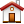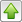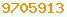# hanging moss algorithm : Java Glossaryhanging moss algorithm
sometimes known as the Spanish moss algorithm. So far as I know, I am the guy that invented it back in the sixties. Somebody will surely point out that Knuth did it first.

Let us say you had N=100,000 points and you wanted to find the closest point to a given point. You could flat-footedly calculate the distance to each point and keep the shortest. If you had to calculate the nearest neighbour to each of your points, the calculation time would be proportional to N² and would become unbearably slow as the number of points increased.

Variants of this problem come up when you try to find the closest point or line segment to plot next to minimise plotter pen motion. It comes up in trying to figure out which country contains a given latitude/longitude. It comes up in trying to rapidly calculate which graphic element in a Canvas a user clicked with a mouse.

The method I am about to describe sounds hideously convoluted, but it is astoundingly faster than doing it the flat-footed way.

You can think of it as a two-dimensional geometric Hashtable.

Define a coarse grid to cover your population of points so that roughly n=10 points fall in each cell and all points fall inside the grid. The actual optimum value of n will be determined later by experiment. Now categorize each of your points by which grid cell they fall in. You can do this with a simple truncated division of the x and y coordinate by the gridsize. Put all the points that fall in the same grid cell, live together on a chain. You just go through all your points categorising them and adding them to the head of the appropriate unidirectional chain for the corresponding grid cell. If you doubly link the chain, you can efficiently delete and insert. If you singly link, (forward or back) you can efficiently do LIFO/FIFO respectively.

The heads of each of the grid chains live in a matrix (array of arrays in Java ).

You can visualise the grid and chain structure as a horizontal grape arbour trellis (the matrix), with chains of Spanish moss dangling down through the grid openings of each cell of the trellis (the chains of points for each cell). This is how the algorithm got its name.

To find the nearest point to (x,y) determine the grid cell that (x,y) falls in. Chase the corresponding chain. Pick the closest point. For some purposes, this may be close enough. To be more accurate, you also need to examine a ring of grid cells around that center cell and take the best point.

If you don’t find any points in that ring, you must examine a ring of grid cells one step further out and compare all the points in the square annulus. Take the best. If you still don’t find any points, go out further till you do, or you exhaust the entire grid.

The scheme works even if you dynamically add or delete points as you go. The hardest part of the implementation is guessing how coarse to make the grid for optimal performance. You really have to do it by experiment.

If you have a few stray points far away from the rest, you can make the code faster by using a grid that covers only the center of the galaxy points, assigning points outside the grid into the nearest perimeter grid cell.

A Hashtable/HashMap works by breaking its big collection of objects to search into subcategories. Then it has to linearly search only one much smaller subcategory. Its way of categorising uses the hash function, followed by taking a modulus. In a Hanging Moss structure, the giant collection of objects is similarly broken into subcategories, but in more intuitive way, by dividing the x and y coordinates to get a pair of ints, which together specify one of the subcategories to linearly search.

Junior Java programmers may be tempted to compute the distance between two points as they learned from Pythagoras:

`dist = Math.sqrt( Math.pow( x2-x1, 2 ) + Math.pow( y2-y1, 2 ));`
Since sqrt is monotonically increasing, you can compute a distance suitable for comparing much more quickly this way, possibly avoiding floating point, (though not 64-bit longs), altogether:
```xdiff = x2-x1;
ydiff = y2-y1;
dist = xdiff*xdiff + ydiff*ydiff;```

There are other implementation issues that are not difficult to deal with, such as encapsulation, Thread safety, coordinate transformation and dealing with the expanding rings banging into the outer edges of the grid. Trying to talk you through them would probably be more confusing than letting you find the obvious solutions for yourself.

According to Mark Thornton, if the set of points to search is constant, a better approach is to use a Voronoi diagram based method (or its dual the Delaunay triangulation). A Voronoi diagram for a set of points can be computed in o(n log(n)) time, then the closest point in the set to any given point can be found in guaranteed log(n) time.

You can also Google on quadtree for other algorithms.

Another trick you can use in these sorts of algorithms is interdigitating. If you have a point with 16-bit coordinates x and y, create a 32-bit single coordinate with even-numbered bits from x and odd-numbered bits from y. If you sort points by this strange number, points near each other in the list will tend to be near each other in space, not quite, but an approximation.

Multipole and fast Multipole math is about ways to rapidly compute pairwise interactions of large numbers of objects.

The advantage of hanging moss over all these more sophisticated mathematic methods is even the most novice programmer can intuitively understand how it works and hence can code it from scratch without help from experts. You also could compile a hanging moss with other algorithms. Hanging moss quickly gets you to the approximate vicinity.This page is postedon the web at: http://mindprod.com/jgloss/hangingmoss.html Optional Replicator mirror of mindprod.com on local hard disk J: J:\mindprod\jgloss\hangingmoss.htmlPlease read the feedback from other visitors, or send your own feedback about the site.Contact Roedy. Please feel free to link to this page without explicit permission. Canadian Mind Products IP:[65.110.21.43] Your face IP:[3.226.251.81] Feedback You are visitor number